# End-to-end visualization using ggplot2

by Vidisha Vachharajani

ggplot2 is kind of a household word for R users. I’ve ended up using it for complex data munging and wrangling work, where I needed to get clarity on different aspects of the data, especially being able to get different views, slices and dices of it, but in a nice visualization. At some point along the line, I slowly stopped using more traditional plotting functions like plot(), matplot(), barplot(), etc.

This article is an end-to-end data visualization exercise, using only ggplot2(). It has been helpful for me to see such pieces online on the endless possibilities of ggplot2(), so I wanted to give back to the community by doing one of my own.

## 1. Pima Indian Diabetes data

Consider the Pima Indian Diabetes dataset available in R. It looks at the population of women who were at least 21 years of age, of Pima Indian heritage and living near Phoenix, Arizona, and were tested for diabetes according to WHO criteria. In this exercise, I will use the 332 test data subjects. There are no missing values in this data. It is a very simple dataset, but my goal is to use it to demonstrate the tools available in ggplot2 to visually investigate a dataset we know very little about. This is part of the important data exploration phase of a data science project, to help prepare for the modeling phase.

library(MASS)
d <- Pima.te
summary(d)
##      npreg             glu              bp              skin
##  Min.   : 0.000   Min.   : 65.0   Min.   : 24.00   Min.   : 7.00
##  1st Qu.: 1.000   1st Qu.: 96.0   1st Qu.: 64.00   1st Qu.:22.00
##  Median : 2.000   Median :112.0   Median : 72.00   Median :29.00
##  Mean   : 3.485   Mean   :119.3   Mean   : 71.65   Mean   :29.16
##  3rd Qu.: 5.000   3rd Qu.:136.2   3rd Qu.: 80.00   3rd Qu.:36.00
##  Max.   :17.000   Max.   :197.0   Max.   :110.00   Max.   :63.00
##       bmi             ped              age         type
##  Min.   :19.40   Min.   :0.0850   Min.   :21.00   No :223
##  1st Qu.:28.18   1st Qu.:0.2660   1st Qu.:23.00   Yes:109
##  Median :32.90   Median :0.4400   Median :27.00
##  Mean   :33.24   Mean   :0.5284   Mean   :31.32
##  3rd Qu.:37.20   3rd Qu.:0.6793   3rd Qu.:37.00
##  Max.   :67.10   Max.   :2.4200   Max.   :81.00
head(d)
##   npreg glu bp skin  bmi   ped age type
## 1     6 148 72   35 33.6 0.627  50  Yes
## 2     1  85 66   29 26.6 0.351  31   No
## 3     1  89 66   23 28.1 0.167  21   No
## 4     3  78 50   32 31.0 0.248  26  Yes
## 5     2 197 70   45 30.5 0.158  53  Yes
## 6     5 166 72   19 25.8 0.587  51  Yes

The target variable, type, tells us whether a patient is diabetic or not.

## 2. Distributions across categories

When the target is categorical, as in this case, type, I like to start by examining distributions for the continuous input columns. This gives us an overall sense of which input is likely to be useful. To do this, I like to do both boxplots and a density plot, since each has a different goal.

### 2.1. Boxplots

First, I’ll use boxplots, but ggplot2-style. I really like the look of a ggplot2() boxplot. It also allows me to seamlessly have multiple plots in a grid, as well as tinker around with the plotting parameters more flexibly than in a classical boxplot() approach, and end up with a nice-looking plot. We can see below how some inputs clearly vary across the 2 target categories, and others don’t.

df <- subset(d, select=c(glu, bp, skin, bmi, ped, age, type))

library(gridExtra)
library(ggplot2)
p <- list()

for (j in colnames(df)[1:6]) {
p[[j]] <- ggplot(data=df, aes_string(x="type", y=j)) + # Specify dataset, input or grouping col name and Y
geom_boxplot(aes(fill=factor(type))) + guides(fill=FALSE) + # Boxplot by which factor + color guide
theme(axis.title.y = element_text(face="bold", size=14))  # Make the Y-axis labels bigger/bolder
}

do.call(grid.arrange, c(p, ncol=3))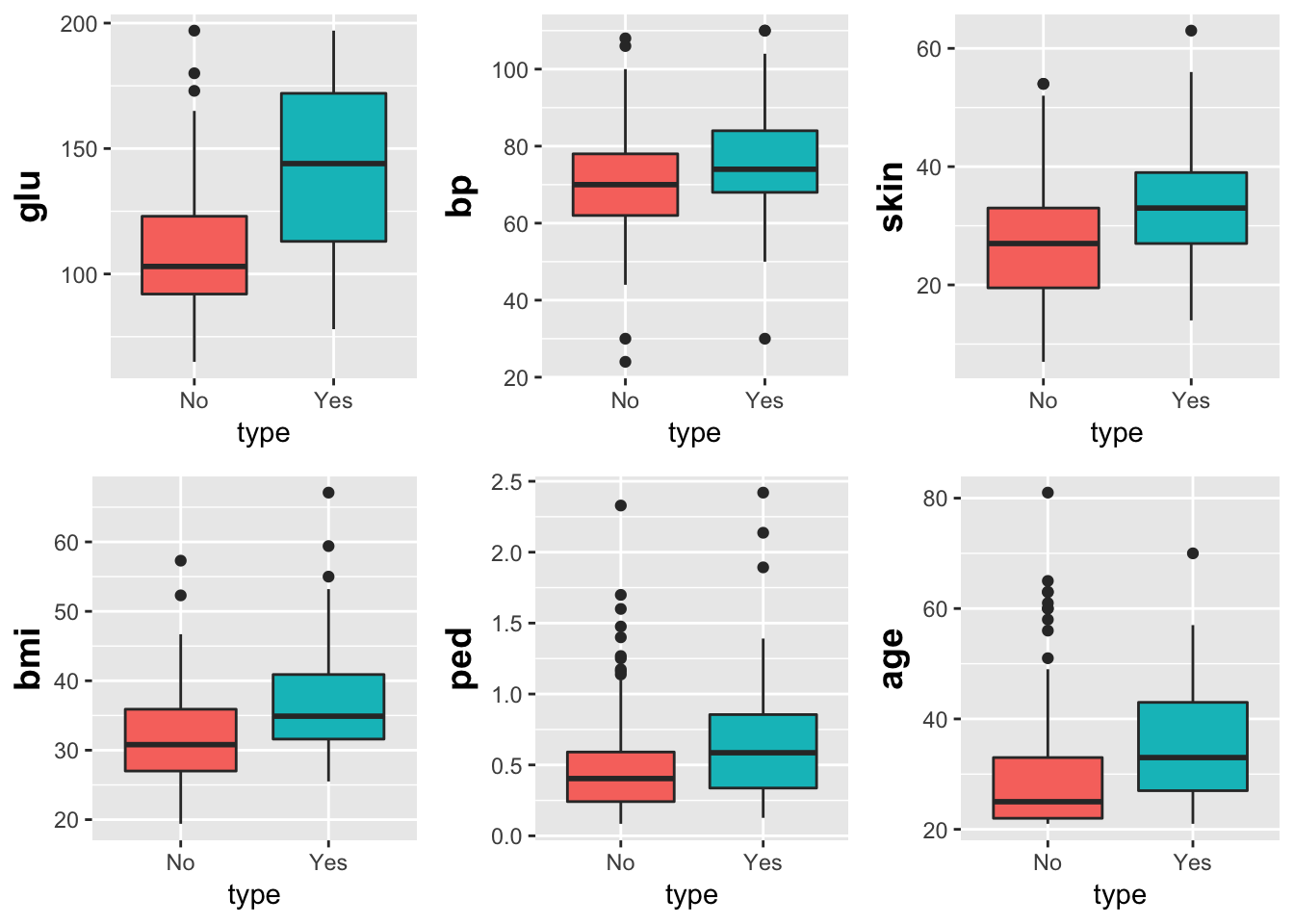### 2.2. Density plots

I have used various overlay-density packages in the past, sm.density.compare() for example. I find the overlay-density rendering in ggplot2() to be more visually pleasing, with little plotting parameter tuning. E.g., it’s clear in the plot below that diabetic patients are associated with more number of pregnancies. I really like the alpha parameter.

df$npreg <- d$npreg
g <- ggplot(df, aes(npreg))
g + geom_density(aes(fill=factor(type)), alpha=0.8) +
labs(title="Density plot",
subtitle="# Pregnancies Grouped by Diabetes Type",
x="# Pregnancies",
fill="Diabetes Type")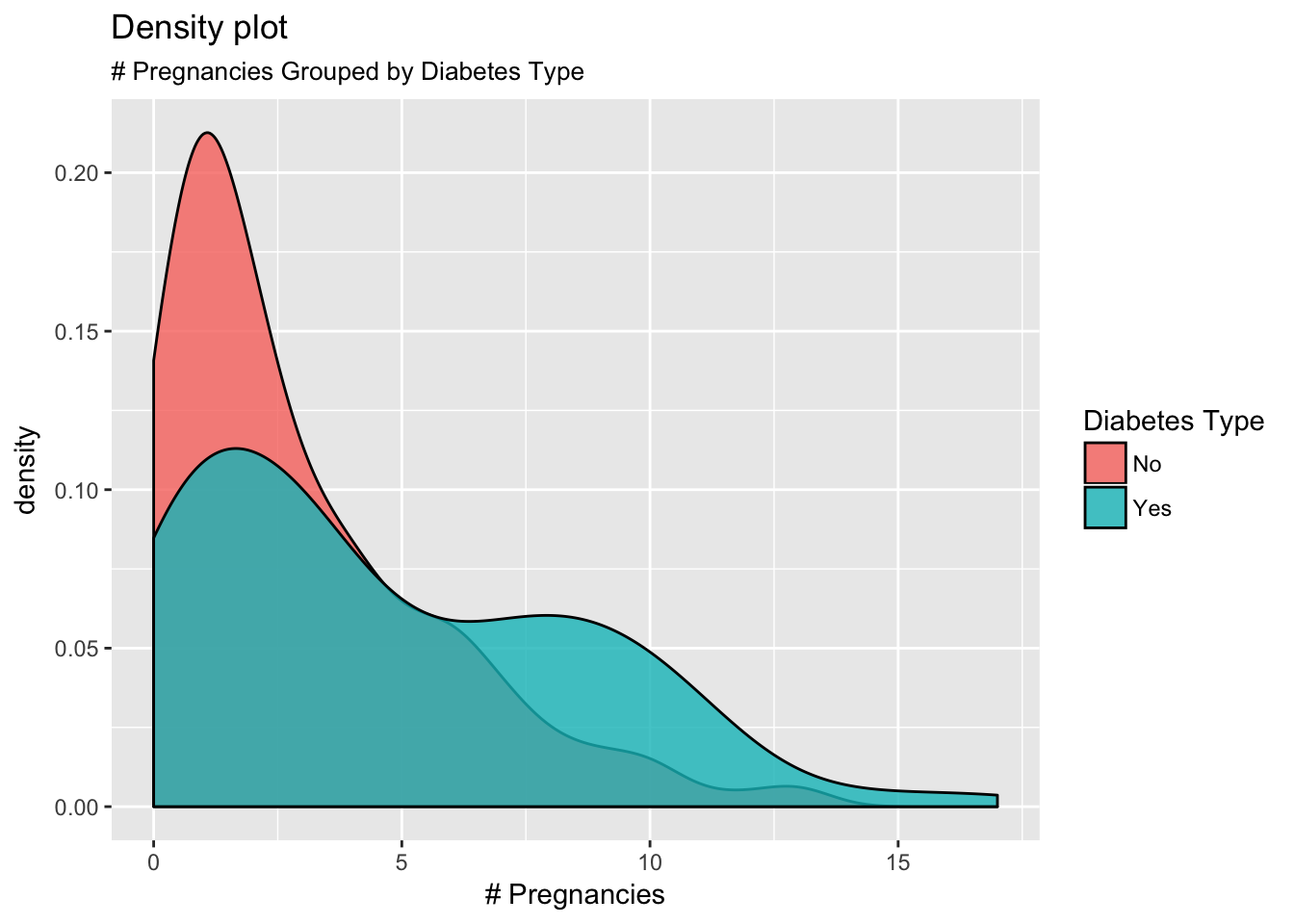## 3. Grid views

Next, I want to mix things up a little, so that I can have multi-dimensional views. By this, I mean that I want to know how the target is distributed across a few important inputs, but I want to link those inputs up as well. Sort of like a 3-way table, but visualized nicely instead of numbers. I came across this problem recently in one of the projects, and while it seems like a basic must-have output to dig deeper, I really needed something like ggplot2 to implement it. Using facet_grid() was amazing, even more so on account of the smooth control one has on the plotting parameters within a ggplot2 setup.

### 3.1. Data preparation

Facet-wrapping and gridding is a must-have tool for deeper data views, but the process is a multi-step one. Not too complicated though - very intuitive under ggplot2. We start with creating some new categorical columns using the continuous ones. Note that this can be done in different ways: appending new columns directly to the data frame, or using the more sleeker dplyr() in combination with magrittr(), which I absolutely love. This integrates a number of operations into a single chunk, making it quite seamless. I am also loading up plyr(), since I will be using it later.

library(magrittr)
library(plyr)
library(dplyr)
df_grid <- d %>%
mutate(Skin = ifelse(d$skin <= 29, "low skin fold", "high skin fold"), BMI = ifelse(d$bmi <= 33, "low BMI", "high BMI"),
Ped = ifelse(d$ped <= 0.31, "low pedigree", ifelse(d$ped > 0.3134 & d$ped <= 0.5844, "medium pedigree", "high pedigree"))) %>% mutate(Ped = factor(Ped, levels = c("low pedigree", "medium pedigree", "high pedigree"))) ### 3.2. Reshaping the data Next, we need to prepare the data a little more before throwing it into the facet_grid() mix. Most importantly, we need to “reshape” it, i.e., while our data is a “wide”-form data frame, we need to convert this to a “long”-form to enable facet_grid() to easily pick up what it needs to “facet” the plot by. We will also add a “size” column - this will allow us to make more granular adjustments in our plot. I will also rename columns in order to enable easier axis labeling when plotting. Again, notice that instead of using reshape2(), which I have used for many years, we’re using gather() from tidyr(), all sewn together with the pipe in magrittr(). library(tidyr) DF <- df_grid %>% subset(select=c(type, Skin, BMI, Ped)) %>% gather(variable, value, -c(Skin, Ped, BMI)) colnames(DF) <- "Diabetes_Value" DF$size <- rep(1.5, nrow(DF))
s <- 1.5

### 3.3. Facet Grid

We’ll try the basic facet_grid() plot, after which we’ll go in and make some adjustments. For now, our goal is the following: to see a “matrix” or “grid” of the BMI distribution across diabetes type, as a 2x2 table of pedigree/skin fold combinations. In other words, for low pedigree/low skin fold, how does BMI distribute across diabetes type? You can see the amount of information you can pack into just one plot. I have found this to be useful when presenting to an end-user or customer. It becomes all the more useful since its a very clear representation of this slice/dice, with little room for ambiguity.

# Simple
library(ggplot2)
ggplot(data=DF, aes(x=Diabetes_Value, fill=BMI)) + geom_bar() +  # Barplot
facet_grid(Skin ~ Ped)   # wrap up everything to showcase by multiple cols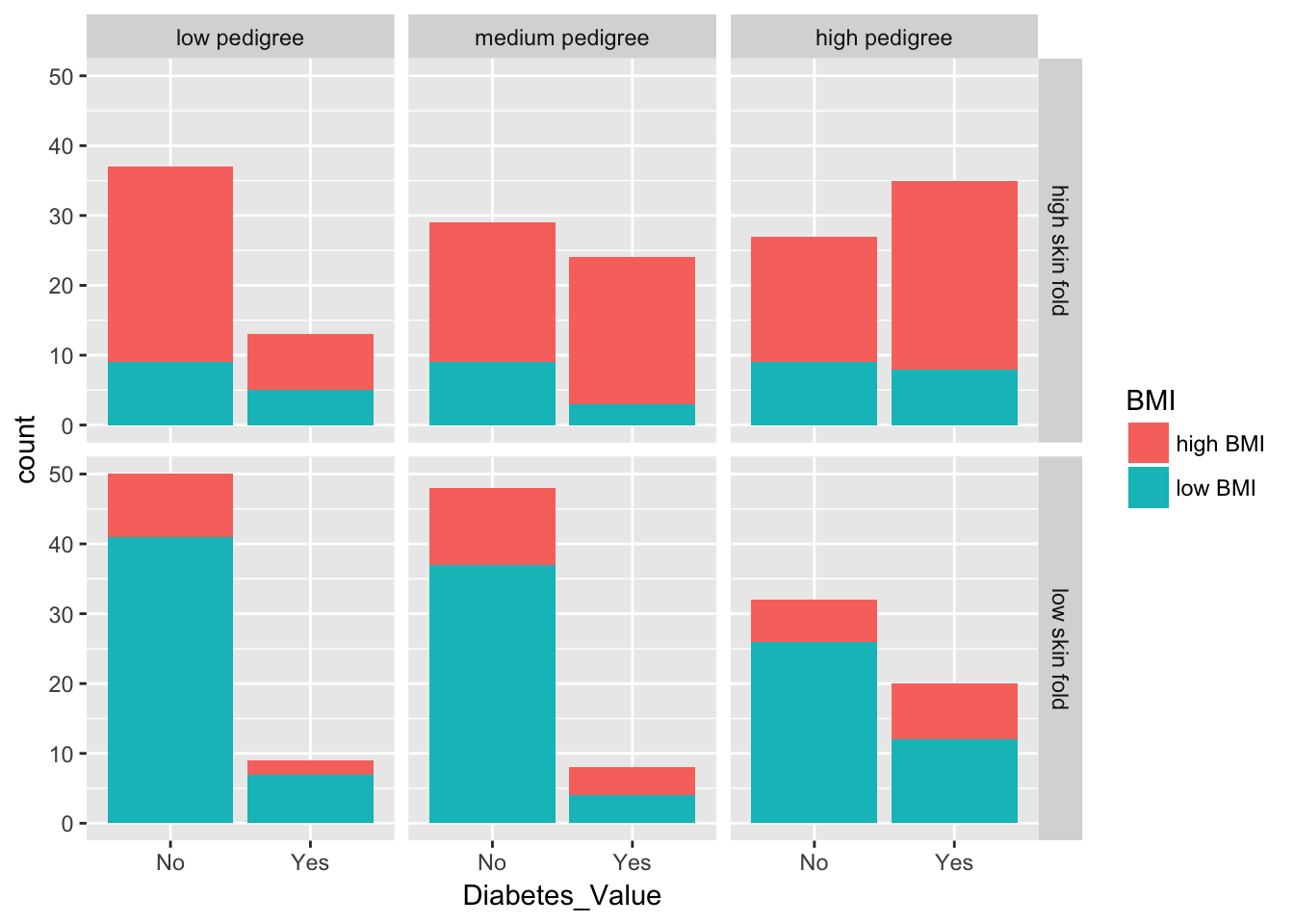This looks nice, but I would like to add more of a “pop”. I am going to outline each box, and bolden the fonts. Note that you can also color the “grid strips”, but I won’t do that right now.

# More color
p <- ggplot(data=DF, aes(x=Diabetes_Value, fill=BMI)) + geom_bar() +  # Barplot
geom_rect(aes(fill=NA, size=size),xmin =-Inf,xmax=Inf,ymin=-Inf,ymax=Inf,alpha = 0.0002, colour="black",show.legend = F) +   # use box drawn around each location to cleanly separate facets + suppress guide
scale_size(range=c(s,s), guide=FALSE) + # use line width/size feature for cleaner plotting
facet_grid(Skin ~ Ped) +   # wrap up everything to showcase by multiple cols
theme(strip.text.x = element_text(face="bold", size=12)) +
theme(strip.text.y = element_text(face="bold", size=12))
# optional changes in strip
#+ theme(strip.text.x = element_text(face="bold", size=12, colour="white")) +
#  theme(strip.text.y = element_text(face="bold", size=12, color="white")) +
#  theme(strip.background = element_rect(fill="black"))
plot(p)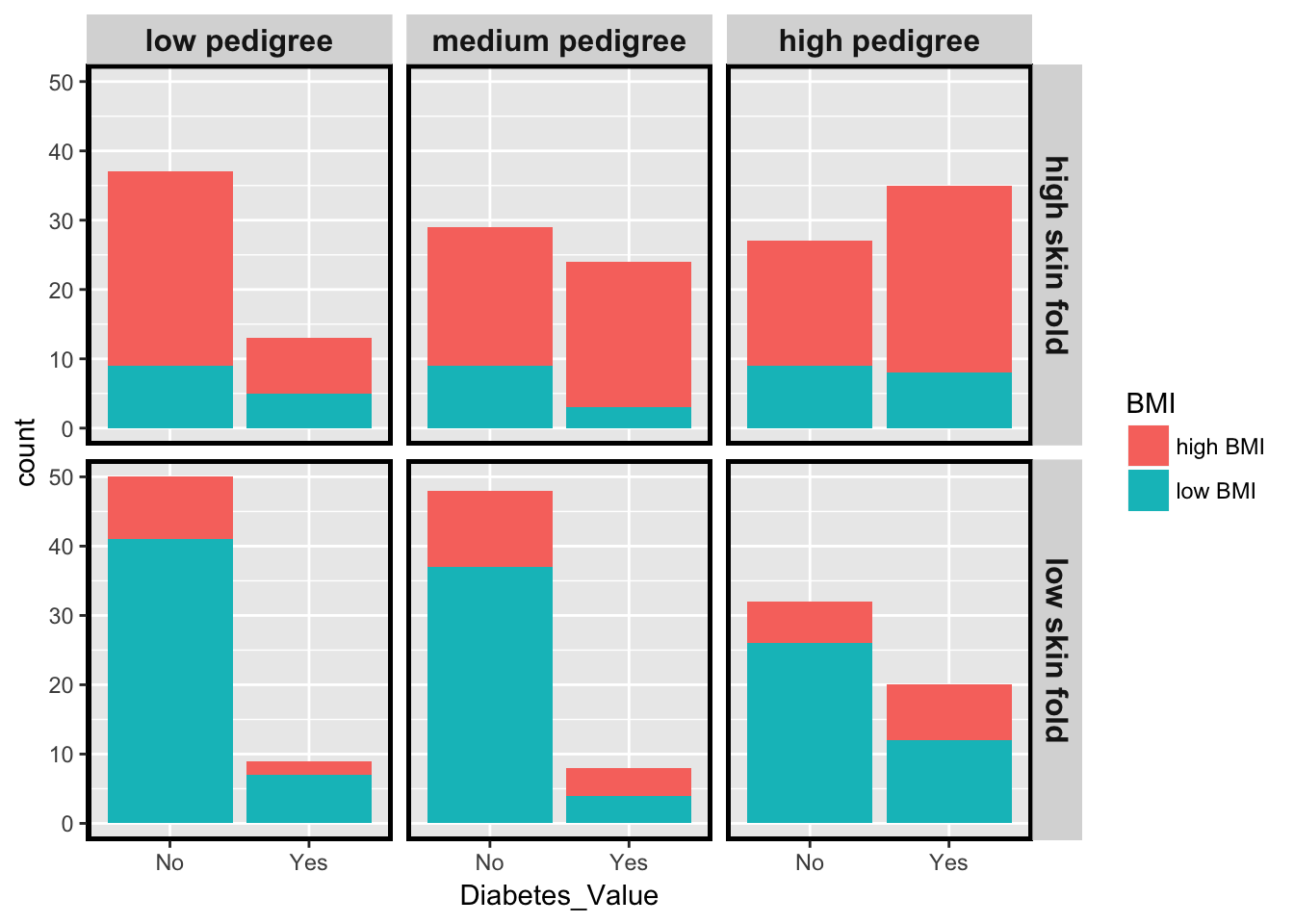Much better. Look how nicely this granular plot adjustment in ggplot2 allows each “block” in the matrix to pop out. Its very clear how BMI is distributed across diabetes type, and how that in turn is distributed across both pedigree function and skin fold. We see that (as expected): 1. A higher triceps skin fold thickness is associated with a higher BMI, as well as a higher count of diabetic people. 2. The above is more true for a higher diabetes pedigree function.

This kind of a grid plot presents a very powerful tool for such multi-dimensional data views.

## 4. Heatmaps

I like heatmaps - there’s a sense of drama in the way you can see where “something is happening”. I’ve used heatmap.2() to implement hierarchical clustering and translating that to a heatmap. But I wanted to use ggplot2() to simply look at a dataset as a heatmap, without any underlying analysis, to detect patterns before any analysis begins.

In this case, I want ggplot2() to show me patterns across different input columns, for the two diabetes types, i.e., what inputs seem to differ across diabetic/non-diabetic patients. This will be clear once we render our dataset into a nice ggplot2() heatmap.

### 4.1. Data preparation

As usual, we need to prep our data before pushing it into the ggplot2() function. We’ll reshape and scale the data first, all within the plyr(), dplyr(), and magrittr() framework. I’ll also specify some plotting parameters that I will call into my ggplot2() function. I’m going to rely on RColorBrewer() for these.

df_heat <- d[order(d$type),1:8] DF_Heat <- df_heat %>% mutate(id = 1:nrow(df_heat)) %>% select(c(npreg:age, id)) %>% gather(variable, value, -id) %>% ddply(.(variable), transform, rescale = scale(value)) # Notice that this reorders by "variables" # Color scale for heatmap library(RColorBrewer) colors <- brewer.pal(9, 'Reds') # Lines to split patients into diabetic/non-diabetic my.lines <- data.frame(x1 = 0.5, x2 = 7.5, y1 = 223.5, y2 = 223.5) ### 4.2. Rendering the heatmap # Basic plot p <- ggplot(DF_Heat, aes(as.factor(variable), as.factor(id), group=id)) + geom_tile(aes(fill = rescale),colour = "white") + scale_fill_gradient(low="green", high="red") # Make adjustments base_size <- 9 p_adj <- p + theme_grey(base_size = base_size) + labs(x = "",y = "") + scale_x_discrete(expand = c(0, 0)) + scale_y_discrete(expand = c(0, 0)) + geom_segment(data=my.lines, aes(x = x1, y = y1, xend=x2, yend=y2), size=1, inherit.aes=F) + theme(axis.text.y = element_blank(), axis.ticks.y = element_blank()) plot(p_adj)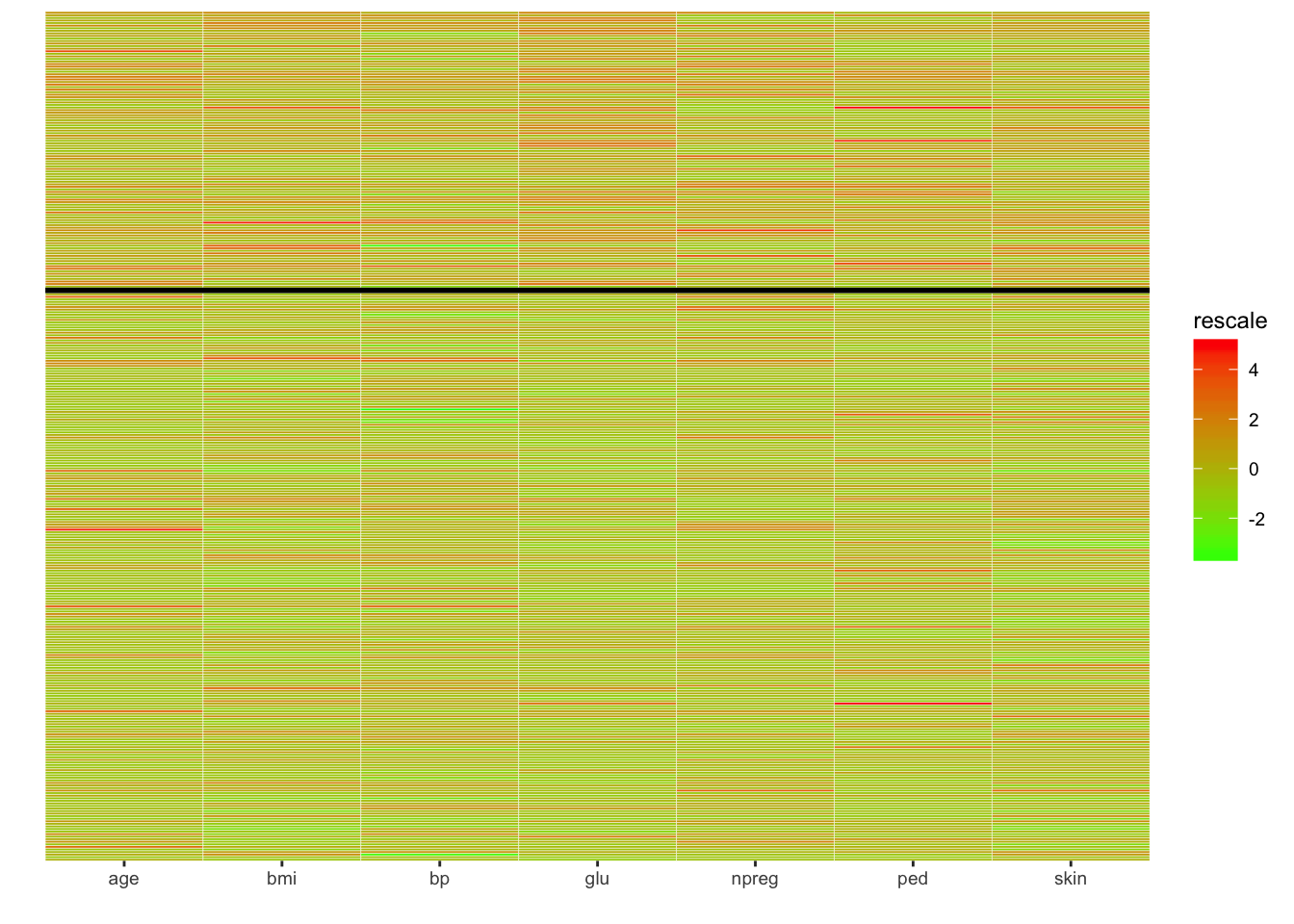Note that I have suppressed the ticks on the Y-axis. We can clearly see regions of interest on the heatmap. It would be better for these to easily pop out at the viewer, to enable which, I am going to invoke geom_rect(). # Borders of rectangles to indicate areas of interest on heatmap my.lines.rect.1 <- data.frame(xmin = 1.5, xmax = 2.5, ymin = 223.5, ymax = 255.5) my.lines.rect.2 <- data.frame(xmin = 3.5, xmax = 4.5, ymin = 223.5, ymax = 332) my.lines.rect.3 <- data.frame(xmin = 5.5, xmax = 6.5, ymin = 223.5, ymax = 280.5) p_adj + geom_rect(data=my.lines.rect.1, aes(xmin = xmin, xmax = xmax, ymin = ymin, ymax = ymax), fill = NA, col = "black", lty=2, inherit.aes = F) + geom_rect(data=my.lines.rect.2, aes(xmin = xmin, xmax = xmax, ymin = ymin, ymax = ymax), fill = NA, col = "black", lty=5, inherit.aes = F) + geom_rect(data=my.lines.rect.3, aes(xmin = xmin, xmax = xmax, ymin = ymin, ymax = ymax), fill = NA, col = "black", lty=4, inherit.aes = F)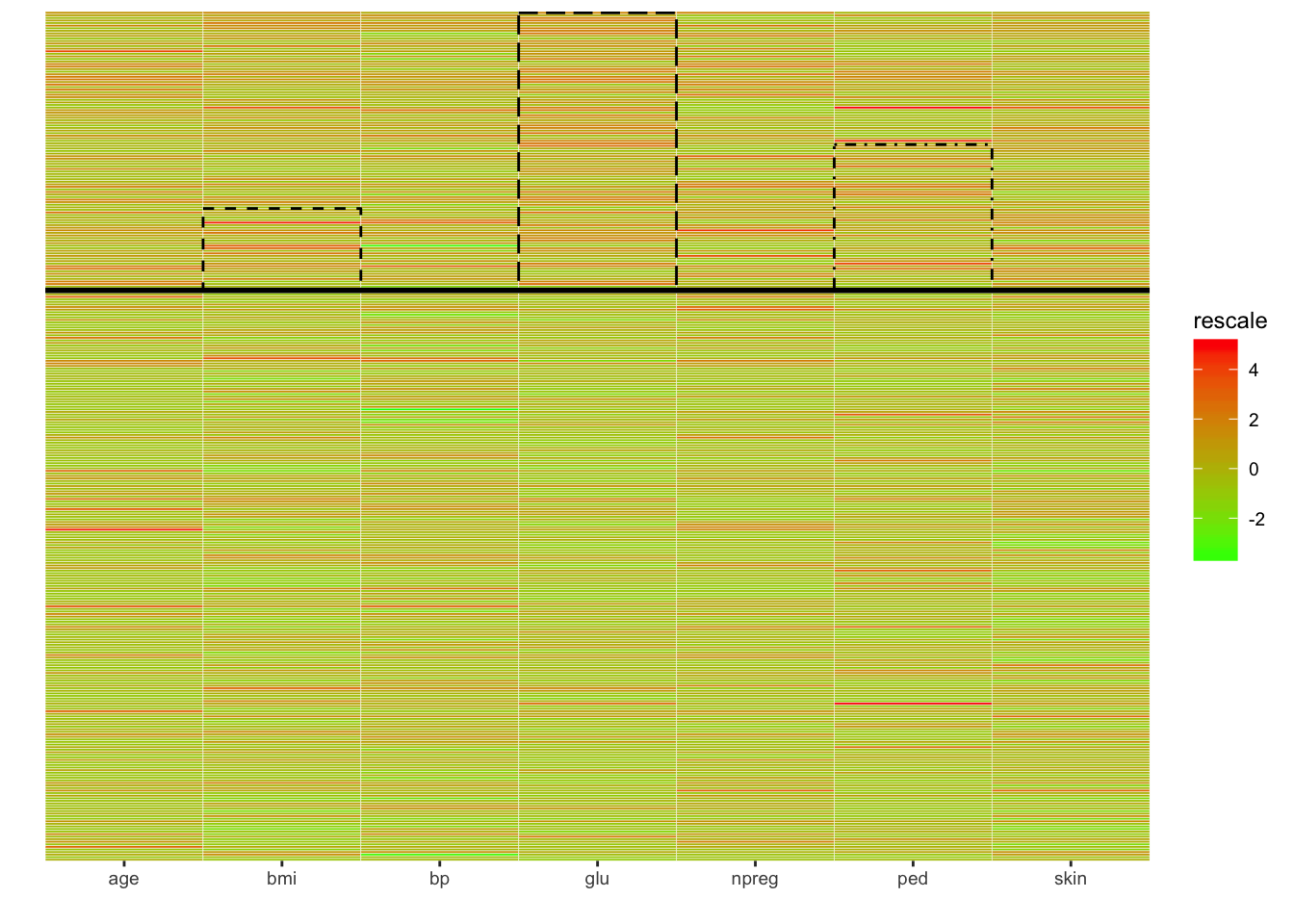Much better. ## 5. Segmentation in a scatterplot Finally, I want to try to implement some “basic-level clustering”. This is not model-based clustering; rather, it is simply using a scatterplot and a few nice plotting parameters in ggplot2() to make some things pop right out at the viewer - again, with little room for ambiguity. What I like most here is the boxes that we can draw nicely to showcase the “clusters” a little better, along-with the multi-layered information, e.g., age, BMI, glucose, etc. The conclusions are logical and obvious from the following plot, but quite nicely illustrate the use of ggplot2() for such a specific purpose. d$Age <- ifelse(d\$age < 30, "<30 yrs", ">= 30 yrs")

ggplot(d, aes(x = glu, y = bmi)) +
geom_rect(aes(linetype = "High BMI - Diabetic"), xmin = 160, ymax = 40, fill = NA, xmax = 200,
ymin = 25, col = "black") +
geom_rect(aes(linetype = "Low BMI - Not Diabetic"), xmin = 0, ymax = 25, fill = NA, xmax = 120,
ymin = 10, col = "black") +
geom_point(aes(col = factor(type), shape = factor(Age)), size = 3) +
scale_color_brewer(name = "Type", palette = "Set1") +
scale_shape(name = "Age") +
scale_linetype_manual(values = c("High BMI - Diabetic" = "dotted", "Low BMI - Not Diabetic" = "dashed"),
name = "Segment")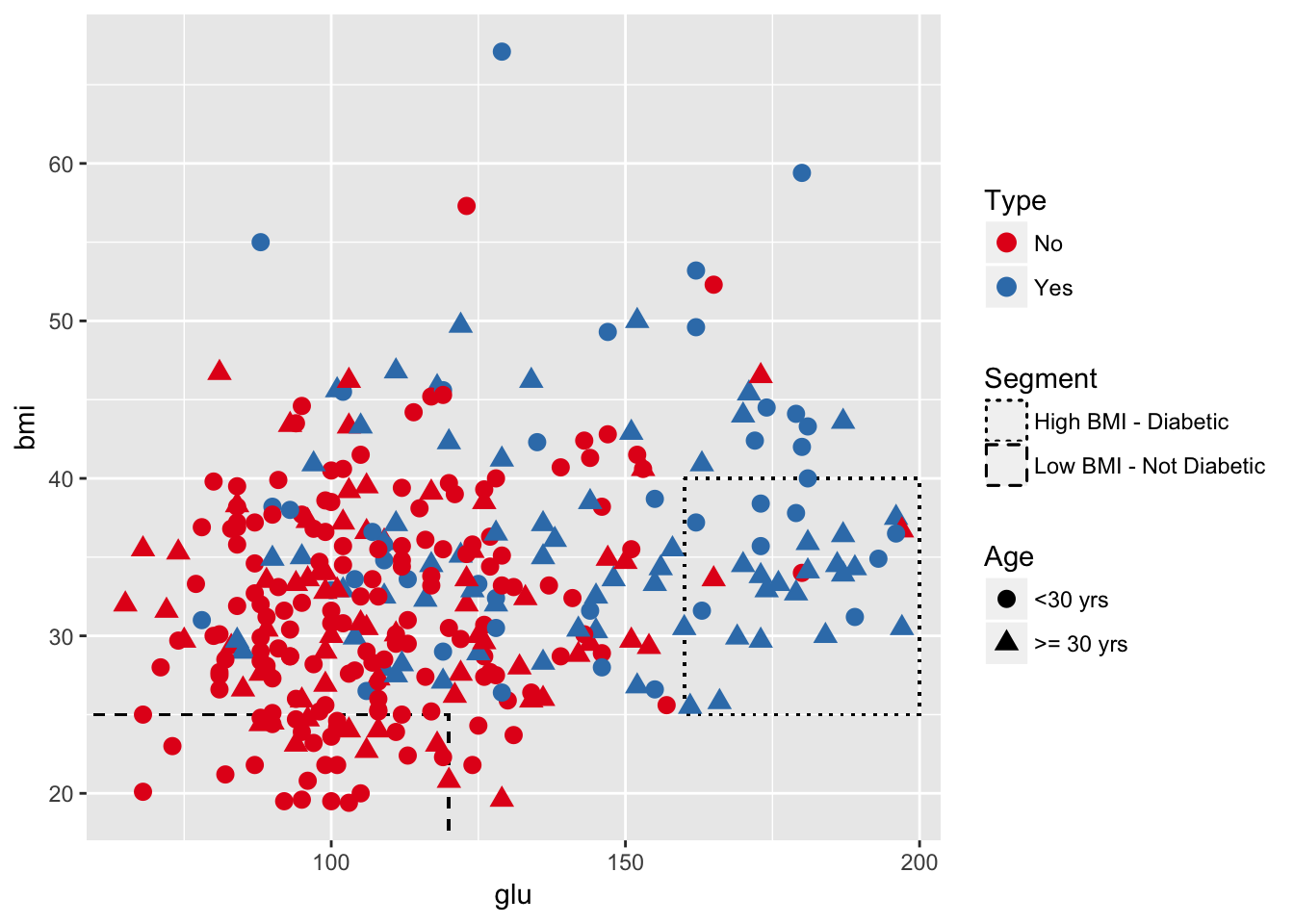Hopefully, this little exercise will be helpful for someone wanting to use ggplot2() for an innovative slice/dice of a complex dataset, and to visualize it nicely.